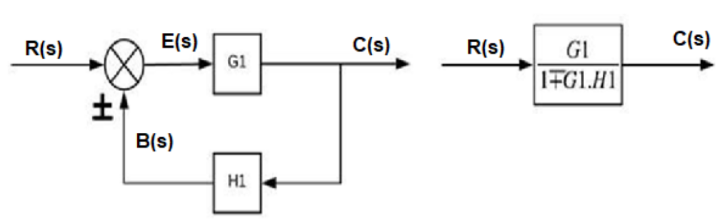### Block Diagram Reduction

Block Diagram Reduction

Block Diagram reduction is used to convert complex systems into simpler systems by following some set of rules in order to deduce the transfer functions easily.

Blocks in Series:
Overall transfer function of all the blocks in series is the multiplication of the transfer function of each individual block.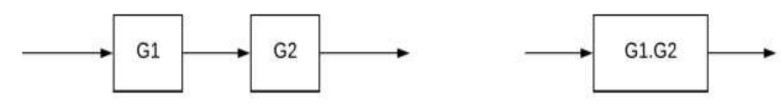Blocks in Parallel:
Transfer function of the whole system will be the addition of the transfer function of each individual block connected in parallel.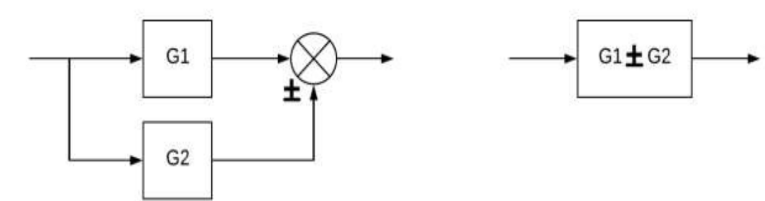Shifting Summing Point After a Block:
When shifting a summing point after a block we have to add a block with same gain in order to get the same output.Shifting Summing Point Before a Block:
When shifting a summing point before a block we have to add a block with inverse(or reciprocal of the original gain) gain to get the original output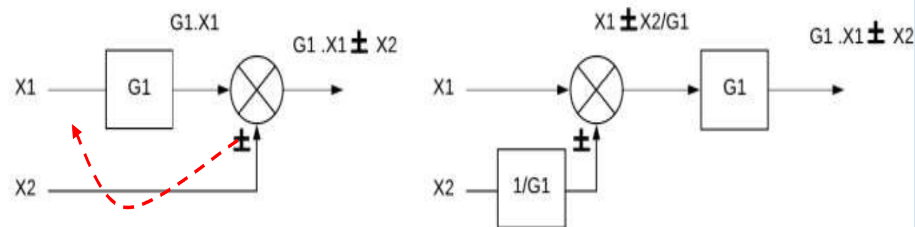Moving takeoff point after a block:
When shifting a takeoff point after a block we have to add a block with inverse (reciprocal of the original gain) gain to get the original output.Moving Takeoff Point Before a Block:
When shifting a summing point after a block we have to add a block with same gain in order to get the same output.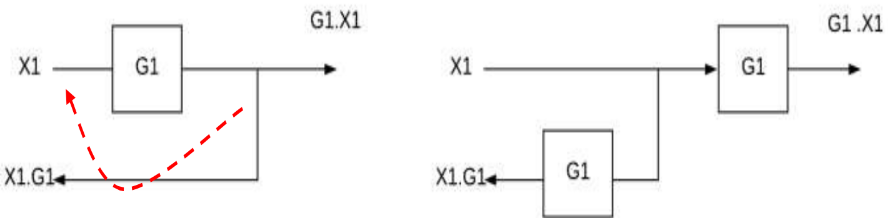Interchange Summing Points:
When 2 summing points are connected in series we can interchange their configuration(order) and the result will still be unchanged.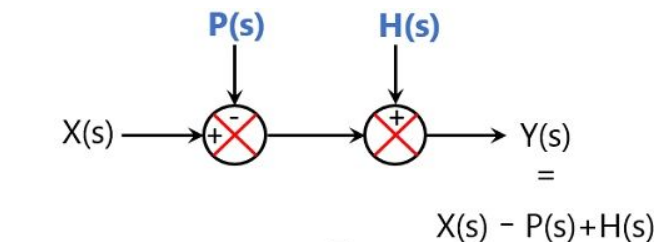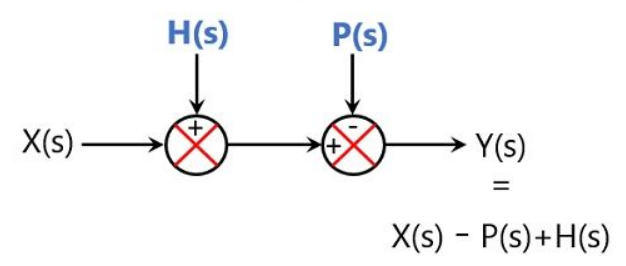Bifurcation of Summing Point with Multiple Inputs into Multiple Summing Points with Single Input:
When there are multiple inputs to a summing point we can split them into multiple single input summing points with different gain inputs.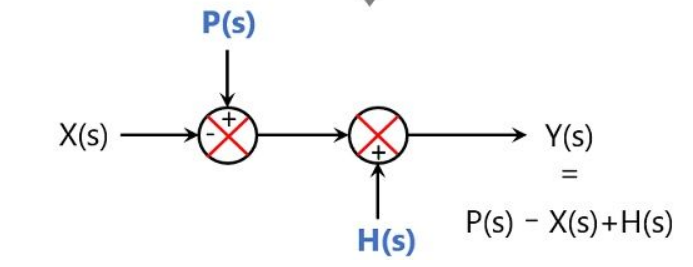Combining Multiple Summing Points into Single Summing Point:
Similarly multiple summing points with single input can be combined together into a single summing point with multiple inputs.Elimination of Feedback Loop:
When there is a feedback loop involved in a system we candeduce it to a single block with feedback gain in forward direction.
Feedback forward gain for negative feedback is given as follows:

Gain =

Where, G is the forward gain and H is the gain of the feedback path.